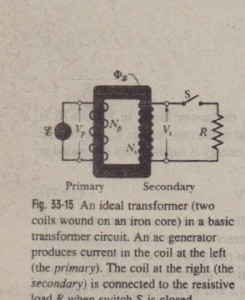# The Ideal Transformer

The Ideal TransformerThe transmission rule leads to a fundamental mismatch between the requirement for efficient high-voltage transmission and the need for safe low-voltage generation and consumption. We need a device with which we can raise (for transmission) and lower (for use) the ac voltage in a circuit, keeping the product current X voltage
essentially constant. The  transformer is such a device. It has no moving parts,operates by Faraday’s law of induction, and has no simple direct-current counterpart The ideal transformer in Fig. 33-15 consists of two coils, with different numbers  of turns, wound around an iron core. (The coils are insulated from the core.) In use,the primary winding, of Np turns, is connected to an alternating-current generator whose em ‘f, at any time t is given by The secondary winding, of N, turns. is connected to load resistance R, but its circui is an open circuit as long as switch S is open (which we assume for the present). Thus, there can be no current through the secondary coil. We assume further for thi  ideal transformer that the resistances of the primary and secondary windings arnegligible, as are energy losses due to magnetic hysteresis in the iron <;are. WelI designed, high-capacity transformers can have energy losses as low as I%. so ou  assumptions are reasonable.For the assumed conditions, the primary winding (or primary) is a pure induc tance, and the primary circuit is like that in Fig. 33-10a. Thus, the (very smalIprimary current, also called the magnetizing current Imag, lags the primary voltage Vp by 90°; the primary’s power factor (= cos c/> in Eq. 33-76) is zero, so no poweris delivered from the generator to the transformer.